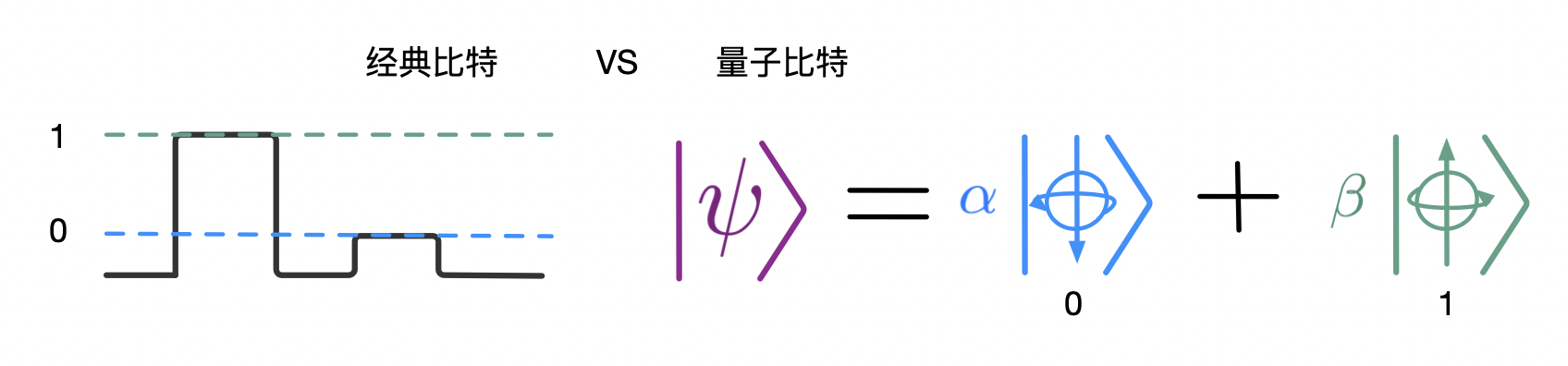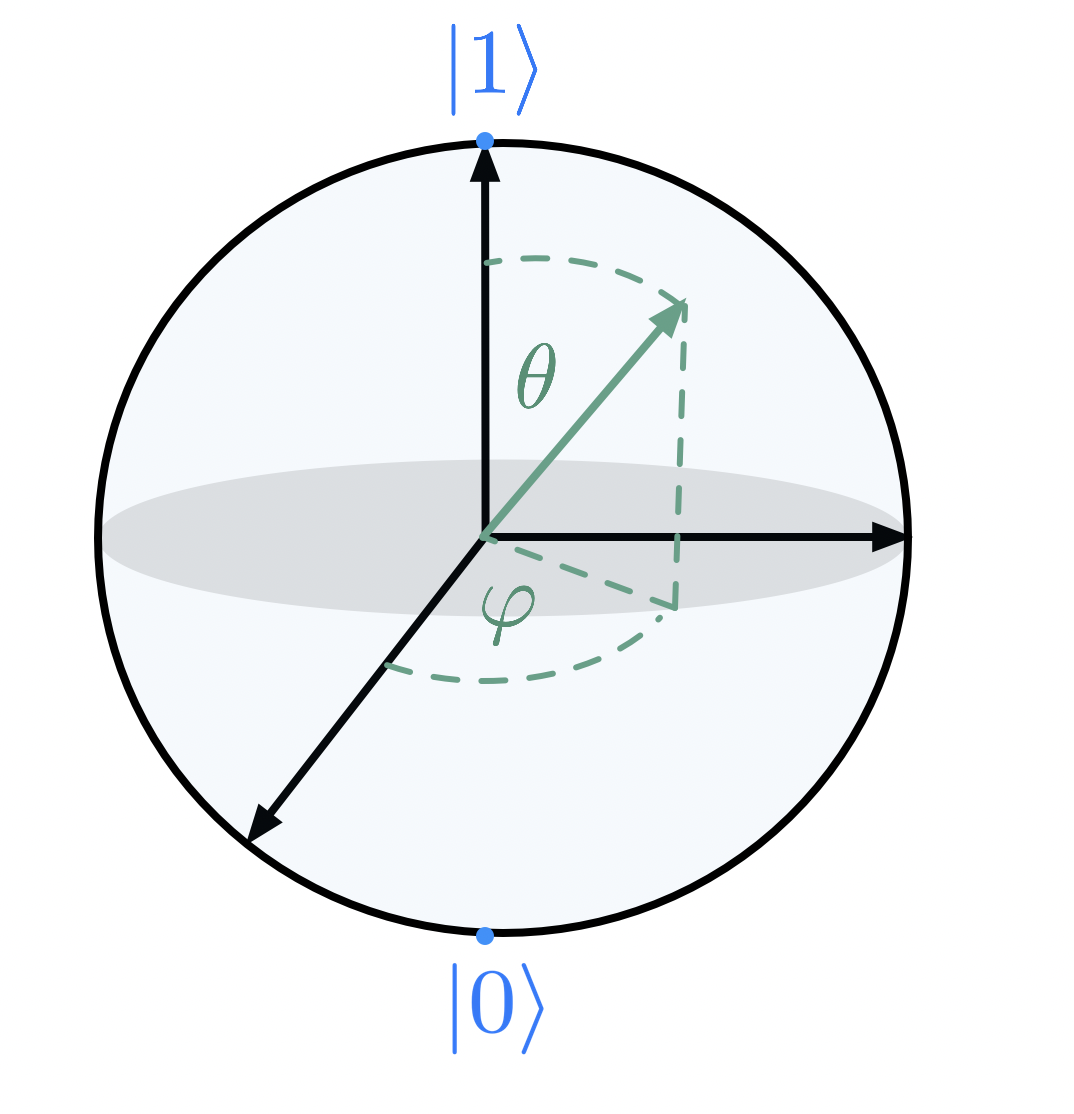# 采采流水

An Essay Concerning Human Understanding

# 量子计算管窥

## 什么是『计算』？

『计算』是一种机械化的信息处理方式。计算过程需要物理实体来承载，所谓机械化指的是计算结果不依赖于计算载体，你可以用手指算，可以用算盘，可以用计算机。阿兰・图灵深得计算本质，提出了图灵机的构想来作为通用计算模型。整个计算的历史堪称一部惊心动魄的史诗，各种人物不时从历史长河中的涌现。这里要提一下，19世纪查尔斯・巴贝奇制造的『差分机』，其它暂且不表。

## 经典计算## 量子计算

$|\psi\rangle = \alpha |0\rangle + \beta|1\rangle.$$|\psi\rangle=\alpha|0\rangle+\beta|1\rangle.$ 两个 Qubit 的话就可以存储4个状态: $|\psi_1\psi_2\rangle = c_{00}|00\rangle+c_{01}|01\rangle+c_{10}|10\rangle+c_{11}|11\rangle=\sum_{i,j=0}^1c_{ij}|ij\rangle.$ 描述 $$n$$ 个 Quibt 需要 $$2^n$$ 个数字，这是一个快速的指数增长。例如 $$n=10$$ ，那就需要大约1024个数来描述所有可能状态。而宇宙中所有原子的总个数也不过是 $$10^{80}$$ 左右的量级，由此可见多个量子比特具有了强大的数字表征能力。

PS：我目前的研究方向就是考察玻色子环境对于量子比特(二能级系统)的影响。此时二能级系统两态之间会有量子隧穿作用，这是让系统演化倾向于量子相干的。此外二能级系统自旋与环境玻色子之间的耦合之后会有一个量子耗散作用，这会使系统倾向于让退相干作用。这两种作用之间存在互相竞争的关系，从而对于这一简单的系统也会存在有趣丰富的物理行为。